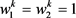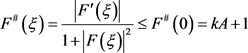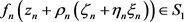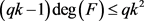﻿ 涉及分担集合的正规族

# 涉及分担集合的正规族The Normal Families of Meromorphic Functions Which Share Set

Abstract: In this paper, it investigates the normality of a family of meromorphic functions sharing a set with their derivatives by using the Nevanlinna's value distribution theory and the method of Zalcman-Pang, and obtains the following main result: Let   be a family of meromorphic functions in a domain D,S1=﹛a1，a2﹜，S2=﹛b1，b2﹜, where S1 and S2 are made up of finite complex numbers, k(≥2) and q be two positive integers. Suppose that for each f∈F , 1) every zero of f-ai is of multiplicities at least k, where i=1,2 ; 2), then   is normal in D.

1. 引言

a是复平面 $ℂ$ 上的一个复数，f和g是区域D内的两个亚纯函数，如果 $f\left(z\right)-a$$g\left(z\right)-a$ 在D内有相同的零点，则称f和g在D内IM分担a；若 $f\left(z\right)-a$$g\left(z\right)-a$ 在D有相同的零点，且所有的零点重级也相等，则称f和g在D内CM分担a。

1996年，方明亮  提出了分担集合的概念。

f和g是区域D内的两个亚纯函数， $S=\left\{{a}_{1},{a}_{2},{a}_{3}\right\}$，S是一个由三个有限复数组成的集合。定义

$\stackrel{¯}{E}\left(S,f\right)=\underset{{a}_{i}\in S}{\cup }\left\{z\in D|f\left(z\right)-{a}_{i}=0\right\}$，其中 $i=1,2,3$。如果 ，则称f和g分担集合S。

$\mathcal{F}$ 为区域D内的一族亚纯函数，我们称 $\mathcal{F}$ 在D内一点 ${z}_{0}$ 正规，如果存在 ${z}_{0}$ 的一个领域 $\Delta \left({z}_{0}\right)$，使 $\mathcal{F}$$\Delta \left({z}_{0}\right)$ 内正规。

1992年，Schwick  最早研究与分担值相关的亚纯函数族的正规性，证明了

2000年，庞学诚和Zalcman  改进了Schwick  的结果，证明了

2002年，方明亮和Zalcman  考虑了f和 ${f}^{\left(k\right)}$ 分担一个值的情形，证明了

2016年，徐焱与仇惠玲  考虑f与 ${f}^{\prime }$ 分担两个集合的亚纯函数的正规性，证明了

2014年，李效敏  等人考虑f和 ${f}^{\left(k\right)}$ 分担集合的情形，证明了

$\ge k$，则 $\mathcal{F}$ 在D内正规。$k\ge 2$ 和q是正整数。显然，对于 $\forall f\in \mathcal{F}$，有 $f={f}^{\left(k\right)}$，而 $\frac{|{f}^{\prime }\left(0\right)|}{1+{|f\left(0\right)|}^{2}}=n\left({w}_{1}-{w}_{2}\right)\to \infty$

2. 引言

1) 函数列 ${f}_{n}\in \mathcal{F}$

2) 点列 ${z}_{n}\in \Delta ,{z}_{n}\to {z}_{0}$

3) 正数列 

4) 实数r， $0

$g\left(\xi \right)$，并且 $g\left(\xi \right)$ 的零点重级 $\ge k$，极点重级 $\ge p$，它的级至多为2， ${g}^{#}\left(\xi \right)=\frac{|{g}^{\prime }\left(\xi \right)|}{1+{|g\left(\xi \right)|}^{2}}\le {g}^{#}\left(0\right)=kA+1$

3. 定理1的证明

${f}_{n}\left({z}_{n}+{\rho }_{n}\zeta \right)-{a}_{1}={g}_{n}\left(\zeta \right)\to g\left(\zeta \right)$ (1)

${f}_{n}\left({z}_{n}+{\rho }_{n}\zeta \right)-{a}_{2}={g}_{n}\left(\zeta \right)+{a}_{1}-{a}_{2}\to g\left(\zeta \right)+{a}_{1}-{a}_{2}$ (2)

${G}_{n}\left(\zeta \right)={\rho }_{n}^{-k}\left({f}_{n}\left({z}_{n}+{\rho }_{n}\zeta \right)-{a}_{1}\right)$。根据已知条件，可知 ${G}_{n}\left(\zeta \right)$ 的零点重级至少为k。取 $\delta \left({\zeta }_{0}\right)>0$，使 ${G}_{n}\left(\zeta \right)$ 在邻域 $\Delta =\left\{\zeta :|\zeta -{\zeta }_{0}|<\delta \left({\zeta }_{0}\right)\right\}$ 内是全纯的，否则， $\left\{{G}_{n}\left({\zeta }_{n}\right)\right\}$$\Delta \left({\zeta }_{0},\delta \right)$ 上内闭一致收敛于全纯函数 $G\left(\zeta \right)$。接下来我们证明 $\left\{{G}_{n}\left(\zeta \right)\right\}$${\zeta }_{0}$ 处不正规。因为 $g\left(\zeta \right)$ 是一个非常数亚纯函数，所以 $g\left(\zeta \right)\overline{)\equiv }0$，则存在一点 ${\zeta }_{0}$，使 $g\left({\zeta }_{0}\right)=0$。根据Hurwitz定理，可知存在一个点列 $\left\{{\zeta }_{n}\right\}\subset \Delta$${\zeta }_{n}\to {\zeta }_{0}$，使 ${g}_{n}\left({\zeta }_{n}\right)={f}_{n}\left({z}_{n}+{\rho }_{n}{\zeta }_{n}\right)-{a}_{1}=0$。当 $\zeta \in {\Delta }^{\prime }=\left\{\zeta :0<|\zeta -{\zeta }_{0}|<\delta \right\}$ 时， $g\left(\zeta \right)\ne 0$。故对任一 $\zeta \in {\Delta }^{\prime }$，存在一个正数 ${\epsilon }_{0}$，使 $|{g}_{n}\left(\zeta \right)|>{\epsilon }_{0}$。从而

$G\left(\zeta \right)=\underset{n\to \infty }{\mathrm{lim}}\frac{{f}_{n}\left({z}_{n}+{\rho }_{n}\zeta \right)-{a}_{1}}{{\rho }_{n}^{k}}\ge \underset{n\to \infty }{\mathrm{lim}}\frac{{\epsilon }_{0}}{{\rho }_{n}^{k}}=\infty$1) 在复平面 $ℂ$$F\left(\xi \right)$ 的零点个数是有限的；

2) $F\left(\xi \right)=0⇔{\left({F}^{\left(k\right)}\left(\xi \right)\right)}^{q}\in {S}_{2}$

${\zeta }_{0}$$g\left(\zeta \right)$ 的l重零点。若 $F\left(\xi \right)$ 零点个数是无穷多个，可取 $F\left(\xi \right)$$l+1$ 个互不相同的零点 ${\xi }_{1},{\xi }_{2},\cdots ,{\xi }_{l+1}$。由于 $F\left(\xi \right)\overline{)\equiv }0$，根据Hurwitz定理，可得存在 ${\xi }_{{n}_{j}}\to {\xi }_{j}\left(j=1,2,\cdots ,l+1\right)$，使得 ${F}_{n}\left({\xi }_{{n}_{j}}\right)=0$，也就是说，当 ${\zeta }_{n}+{\eta }_{n}{\xi }_{{n}_{j}}\to {\zeta }_{0}$ 时， ${f}_{n}\left({z}_{n}+{\rho }_{n}\left({\zeta }_{n}+{\eta }_{n}{\xi }_{{n}_{j}}\right)\right)-{a}_{1}=0$。根据Hurwitz定理， ${\zeta }_{0}$$g\left(\zeta \right)$ 的至少 $l+1$ 重零点，矛盾。故断言1)成立。

$F\left({\xi }_{0}\right)=0$，由Hurwitz定理，可知存在一点列 ${\xi }_{n}\to {\xi }_{0}$，使得 ${f}_{n}\left({z}_{n}+{\rho }_{n}\left({\zeta }_{n}+{\eta }_{n}{\xi }_{n}\right)\right)={a}_{1}$。由条件可得

${\left({F}_{n}^{\left(k\right)}\left({\xi }_{n}\right)\right)}^{q}={\left({f}_{n}^{\left(k\right)}\left({z}_{n}+{\rho }_{n}\left({\zeta }_{n}+{\eta }_{n}{\xi }_{n}\right)\right)\right)}^{q}\in {S}_{2}$

$\underset{n\to \infty }{\mathrm{lim}}{\left({F}_{n}^{\left(k\right)}\left({\xi }_{n}\right)\right)}^{q}={\left({F}^{\left(k\right)}\left({\xi }_{0}\right)\right)}^{q}\in {S}_{2}$。因此证明了 $F\left(\xi \right)=0⇒{\left({F}^{\left(k\right)}\left(\xi \right)\right)}^{q}\in {S}_{2}$

${\left({F}^{\left(k\right)}\left(\xi \right)\right)}^{q}\equiv {b}_{i}$，则 $F\left(\xi \right)$ 是一个次数至多为k的多项式。因为 $F\left(\xi \right)$ 的零点重级 $\ge k$，可得 $F\left(\xi \right)$ 是一个

${b}_{i}\ne 0\left(i=1,2\right)$ 时，

${F}^{#}\left(0\right)=\frac{|{F}^{\prime }\left(0\right)|}{1+{|F\left(0\right)|}^{2}}=\frac{\frac{\sqrt[q]{|{b}_{i}|}}{\left(k-1\right)!}{|{\xi }_{0}|}^{k-1}}{1+\frac{{\left(\sqrt[q]{|{b}_{i}|}\right)}^{2}}{{\left(k!\right)}^{2}}{|{\xi }_{0}|}^{2k}}$

${F}^{#}\left(0\right)\le \left\{\begin{array}{l}\frac{\sqrt[q]{|{b}_{i}|}}{\left(k-1\right)!}{|{\xi }_{0}|}^{k-1}\le \frac{\sqrt[q]{|{b}_{i}|}}{2},\text{\hspace{0.17em}}\text{\hspace{0.17em}}\text{\hspace{0.17em}}|{\xi }_{0}|<1\\ \frac{\frac{\sqrt[q]{|{b}_{i}|}}{\left(k-1\right)!}{|{\xi }_{0}|}^{k-1}}{2\frac{\sqrt[q]{|{b}_{i}|}}{k!}{|{\xi }_{0}|}^{k}}\le \frac{k}{2},\text{\hspace{0.17em}}\text{\hspace{0.17em}}\text{\hspace{0.17em}}\text{\hspace{0.17em}}\text{\hspace{0.17em}}\text{\hspace{0.17em}}\text{ }\text{ }|{\xi }_{0}|\ge 1\end{array}$

${\left({F}_{n}^{\left(k\right)}\left({\xi }_{n}\right)\right)}^{q}={\left({f}_{n}^{\left(k\right)}\left({z}_{n}+{\rho }_{n}\left({\zeta }_{n}+{\eta }_{n}{\xi }_{n}\right)\right)\right)}^{q}={b}_{i}$。根据条件可得，。可证，当 $n\to \infty$

$F\left({\xi }_{0}\right)=\underset{n\to \infty }{\mathrm{lim}}{\rho }_{n}^{-k}{\eta }_{n}^{-k}\left({f}_{n}\left({z}_{n}+{\rho }_{n}\left({\zeta }_{n}+{\eta }_{n}{\xi }_{n}\right)\right)-{a}_{1}\right)=\underset{n\to \infty }{\mathrm{lim}}{\rho }_{n}^{-k}{\eta }_{n}^{-k}\left({a}_{2}-{a}_{1}\right)=\infty$

$T\left(r,{\left({F}^{\left(k\right)}\right)}^{q}\right)\le \underset{i=1}{\overset{2}{\sum }}\stackrel{¯}{N}\left(r,\frac{1}{{\left({F}^{\left(k\right)}\right)}^{q}-{b}_{i}}\right)+\stackrel{¯}{N}\left(r,F\right)+O\left(1\right)\le \stackrel{¯}{N}\left(r,\frac{1}{F}\right)+O\left(1\right)$ (3)

$T\left(r,{\left({F}^{\left(k\right)}\right)}^{q}\right)=q\left(\mathrm{deg}\left(F\right)-k\right)\mathrm{log}r+O\left(1\right)$ (4)

$\stackrel{¯}{N}\left(r,\frac{1}{F}\right)\le \frac{N\left(r,\frac{1}{F}\right)}{k}=\frac{T\left(r,F\right)+O\left(1\right)}{k}=\frac{\mathrm{deg}\left(F\right)}{k}\mathrm{log}r+O\left(1\right)$ (5)(6)

$k\le \mathrm{deg}\left(F\right)\le \frac{q{k}^{2}}{qk-1}\le 2k$ (7)

$F\left(\xi \right)={c}_{0}{\left(\xi -{\xi }_{1}\right)}^{\mathrm{deg}\left(F\right)}$，其中 ${c}_{0}$ 为非零常数，对任意的 $i\left(i=1,2\right)$${\left({F}^{\left(k\right)}\left(\xi \right)\right)}^{q}-{b}_{i}$ 至少有一个零点。根据断言2)，可知 $F\left(\xi \right)$ 至少有两个不同的零点。

$P=\frac{F{F}^{\left(3\right)}}{\left({F}^{\left(2\right)}-{b}_{1}\right)\left({F}^{\left(2\right)}-{b}_{2}\right)}$ (8)

$F\left(\xi \right)={a}_{m}{\xi }^{m}+{a}_{m-1}{\xi }^{m-1}+\cdots +{a}_{1}\xi +{a}_{0}+\frac{{P}_{1}\left(\xi \right)}{{P}_{2}\left(\xi \right)}$ (9)

${P}_{2}\left(\xi \right)=\mu {\left(\xi -{\xi }_{1}\right)}^{{m}_{1}}{\left(\xi -{\xi }_{2}\right)}^{{m}_{2}}\cdots {\left(\xi -{\xi }_{t}\right)}^{{m}_{t}}$ (10)

$N\left(r,{\left({F}^{\left(k\right)}\right)}^{q}\right)=q\left(w+kt\right)\mathrm{log}r+O\left(1\right)$ (11)

$\stackrel{¯}{N}\left(r,F\right)=\stackrel{¯}{N}\left(r,{\left({F}^{\left(k\right)}\right)}^{q}\right)=t\mathrm{log}r+O\left(1\right)$ (12)

$\begin{array}{c}T\left(r,{\left({F}^{\left(k\right)}\right)}^{q}\right)\le \stackrel{¯}{N}\left(r,F\right)+\stackrel{¯}{N}\left(r,\frac{1}{F}\right)+O\left(1\right)\\ \le q\left(\stackrel{¯}{N}\left(r,F\right)+\stackrel{¯}{N}\left(r,\frac{1}{F}\right)\right)+O\left(1\right)\end{array}$ (13)

$\stackrel{¯}{N}\left(r,\frac{1}{F}\right)\le \frac{1}{k}N\left(r,\frac{1}{F}\right)=\frac{m+w}{k}\mathrm{log}r+O\left(1\right)\le \frac{m+w}{2}\mathrm{log}r+O\left(1\right)$ (14)

$\begin{array}{c}T\left(r,{\left({F}^{\left(k\right)}\right)}^{q}\right)=N\left(r,\frac{1}{{\left({F}^{\left(k\right)}\right)}^{q}}\right)+O\left(1\right)\\ =q\left[\left(m-k\right)+\left(w+kt\right)\right]\mathrm{log}r+O\left(1\right)\\ =q\left[m+w+\left(t-1\right)k\right]\mathrm{log}r+O\left(1\right)\end{array}$ (15)

$\frac{m+w}{2}\le -k\left(t-1\right)+t\le 2-t$ . (16)

$T\left(r,{\left({F}^{\left(k\right)}\right)}^{q}\right)=N\left(r,{\left({F}^{\left(k\right)}\right)}^{q}\right)+O\left(1\right)=q\left(w+kt\right)\mathrm{log}r+O\left(1\right)$

$q\left(w+kt\right)\mathrm{log}r\le q\left(t+\frac{m+w}{k}\right)\mathrm{log}r+O\left(1\right)$，因此 $k<\left(k-1\right)w+\left(k-1\right)kt\le m 矛盾。(17)

$g\left(\zeta \right)+{a}_{1}-{a}_{2}\ne 0$，可得 ${\left({g}^{\left(k\right)}\left(\zeta \right)\right)}^{q}\ne 0$，即 ${g}^{\left(k\right)}\left(\zeta \right)\ne 0$

$T\left(r,g\right)=\stackrel{¯}{N}\left(r,g\right)+\stackrel{¯}{N}\left(r,\frac{1}{g}\right)+\stackrel{¯}{N}\left(r,\frac{1}{g+{a}_{1}-{a}_{2}}\right)+O\left(\mathrm{log}r\right) (18)

 Gu, Y.X., Fang, M.L. and Pang, X.C. (2007) The Theory of Normal Families and It’s Applications. Science Press, Beijing.

 Ahlfors, L.V. (1979) Complex Analysis. 3rd Edition, McGraw-Hill, Hoboken.

 Schiff, J. (1993) Normal Families. Springer-Verlag, Berlin.
https://doi.org/10.1007/978-1-4612-0907-2

 Xu, J.F. and Cao, W.S. (2010) Some Normality Criteria of Meromorphic Functions. Journal of Inequalities and Applications, 2010, Article ID: 926302.
https://doi.org/10.1155/2010/926302

 Chen, H.H., Cai, J.H. and Yuan, W.J. (2018) Normality Criteria of a Family of Meromorphic Functions Concerning Shared Set. Journal of Jiaying University, 36, 5-8.

 Jiang, Y.B. and Gao, Z.S. (2011) Normal Families of Meromorphic Functions Sharing Values or Functions. Journal of Inequalities and Applications, 2011, Article No. 72.
https://doi.org/10.1186/1029-242X-2011-72

 Qiu, L. and Hu, F.F. (2013) Normal Families of Meromorphic Functions Sharing One Function. Journal of Inequalities and Applications, 2013, 288.
https://doi.org/10.1186/1029-242X-2013-288

 Fang, M.L. (1996) A Note on Sharing Values and Normality. Journal of Mathematical Study, 29, 29-32.

 Schwick, W. (1992) Sharing Values and Normality. Archiv der Mathematik, 59, 50-54.
https://doi.org/10.1007/BF01199014

 Pang, X.C. and Zalcman, L. (2000) Normality and Shared Values. Arkiv för Matematik, 38, 171-182.
https://doi.org/10.1007/BF02384496

 Liu, X.J. and Pang, X.C. (2007) Shared Values and Normality. Acta Mathematica Sinica, 52, 409-412.

 Fang, M.L. and Zalcman, L. (2002) Normal Families and Shared Values of Meromorphic Functions. Computational Methods and Function Theory, 2, 385-395.
https://doi.org/10.1007/BF03321856

 Zhang, H., Xie, D. and Zhang, Q.D. (2011) Normal Criteria Concerning Shared Values of Meromorphic Function. Acta Mathematica Scientia A, 31, 1290-1294.

 Xu, Y. and Qiu, H.L. (2016) Normal Functions and Shared Sets. Filomat, 30, 287-292.
https://doi.org/10.2298/FIL1602287X

 Li, X.M., Yi, H.X. and Wang, K.M. (2014) Notes on Normal Families of Meromorphic Functions Sharing a Set with Their Derivatives. Bulletin of the Korean Mathematical Society, 51, 1773-1789.
https://doi.org/10.4134/BKMS.2014.51.6.1773

 Pang, X.C. and Zalcman, L. (2000) Normal Families and Shared Values. Bulletin of the London Mathematical Society, 32, 325-331.
https://doi.org/10.1112/S002460939900644X

 Bergweiler, W. and Eremenko, A. (1995) On the Singularities of the Inverse to a Meromorphic Functions of Finite Order. Revista Matemática Iberoamericana, 11, 355-372.
https://doi.org/10.4171/RMI/176

 Zalcman, L. (1998) Normal Families: New Perspective. Bulletin of the American Mathematical Society, 35, 215-230.
https://doi.org/10.1090/S0273-0979-98-00755-1

 Yang, L. (1982) Value Distribution Theory and It’s New Research. Science Press, Beijing.

 Langley, J.K. (2003) The Second Derivative of a Meromorphic Function of Finite Order. Bulletin of the London Mathematical Society, 35, 97-108.
https://doi.org/10.1112/S0024609302001558

Top